﻿ 云南地区生产总值影响因素和回归分析 Influencing Factors and Regres-sion Analysis of GDP in Yunnan Province

Statistics and Application
Vol. 08  No. 04 ( 2019 ), Article ID: 31573 , 8 pages
10.12677/SA.2019.84066

Influencing Factors and Regression Analysis of GDP in Yunnan Province

Xinxin Hu

School of Statistics and Mathematics, Yunnan University of Finance and Economics University, Kunming Yunnan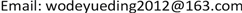Received: Jun. 27th, 2019; accepted: Jul. 13th, 2019; published: Aug. 2nd, 2019ABSTRACT

Based on the statistical yearbook of GDP from 2007 to 2016 in Yunnan province and the related data, using linear regression method, this paper sets up the fitting model to describe the relationship between GDP and related variables in Yunnan province. The heteroscedasticity test, sequence autocorrelation test and abnormal point test for the model are also carried out. The results show that this model can be used to predict the gross domestic product of Yunnan province.

Keywords:GDP, Influencing Factors, Linear Regression Model1. 引言

2. 数据来源与变量选择

2.1. 数据来源

2.2. 变量选择

3. 建立模型

3.1. 模型估计 

3.2. 用普通最小二乘法(OLS)估计模型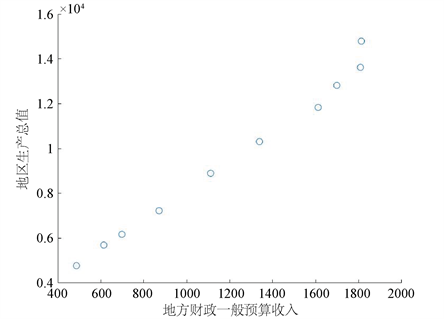(1)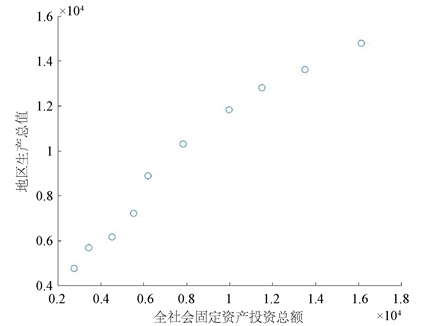(2)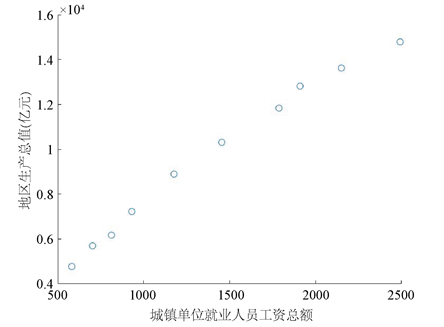(3)(4)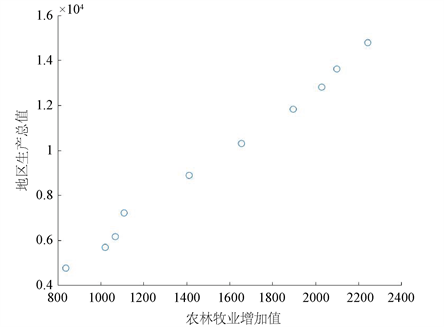(5)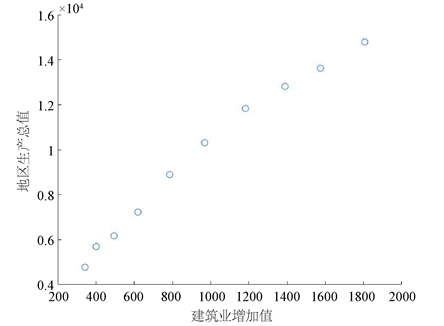(6)(7)

Figure 1. The scatter plot between the variable Xi ( $i=0,\cdots ,7$ ) and Y((1)~(7))

$Y={\beta }_{0}+{\beta }_{1}{X}_{1}+{\beta }_{2}{X}_{2}+{\beta }_{3}{X}_{3}+{\beta }_{4}{X}_{4}+{\beta }_{5}{X}_{5}+{\beta }_{6}{X}_{6}+{\beta }_{7}{X}_{7}+\mu$

$hY=-592.094-1.004{X}_{1}-0.038{X}_{2}-1.586{X}_{3}+1.440{X}_{4}+2.272{X}_{5}+4.253{X}_{6}+16.519{X}_{7}+\mu$Table 2. System resulting data of standard experiment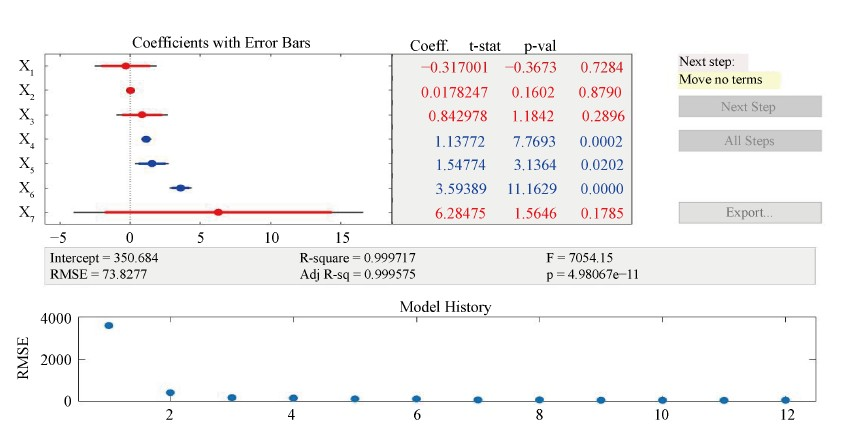Figure 2. The result of stepwise

3.3. 异方差性检验

a. 残差图分析法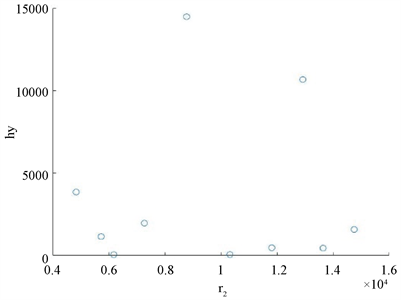Figure 3. The residual plot

b. 斯皮尔曼(Spearman)检验 

4. 结论

Influencing Factors and Regres-sion Analysis of GDP in Yunnan Province[J]. 统计学与应用, 2019, 08(04): 581-588. https://doi.org/10.12677/SA.2019.84066

1. 1. 陈静. 我国各地区生产总值的影响因素分析及建议[J]. 商, 2015(7): 150-151.

2. 2. 杨武. 安徽省国内生产总值影响因素的多元回归分析[J]. 南方农机, 2018, 49(5): 11-13.

3. 3. 李实. 云南省生产总值影响因素实证分析[J]. 中国市场, 2011(31): 105-107.

4. 4. 朱琳, 陈飞. 云南失业率影响因素分析和回归诊断[J]. 当代经济, 2013(4): 90-91.

5. 5. 王雪雪. 我国地区生产总值影响因素的实证分析[J]. 时代金融, 2017(15): 18-22.

6. 6. 单翔翔, 严浩坤. 基于多元回归模型分析我国国内生产总值的影响因素[J]. 时代金融, 2018(9): 238-239.

7. 7. 吴喜之. 应用回归及分类[M]. 北京: 中国人民大学出版社, 2016: 49-51.

8. 8. 王燕. 应用时间序列[M]. 北京: 中国人民大学出版社, 2012: 82-83.

9. 9. 唐年胜, 李会琼. 应用回归分析[M]. 北京: 科学出版社, 2014: 114-115.

10. 10. 杨林涛. 非参数统计视角下的异方差检验设计及其应用[J]. 数量经济技术经济研究, 2014, 31(11): 118-131.